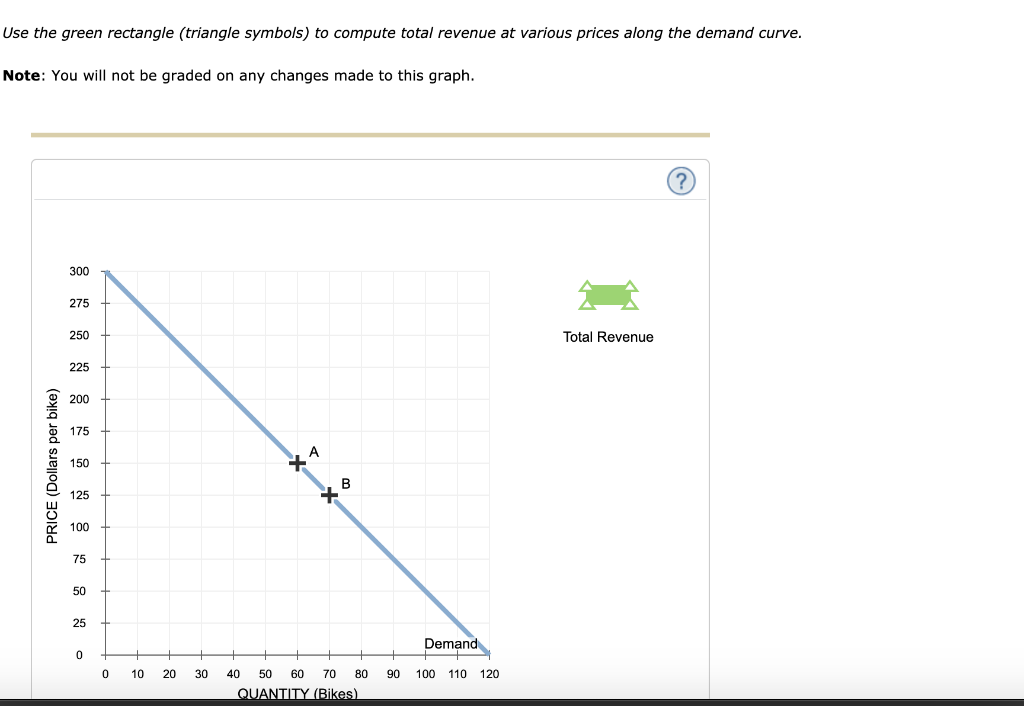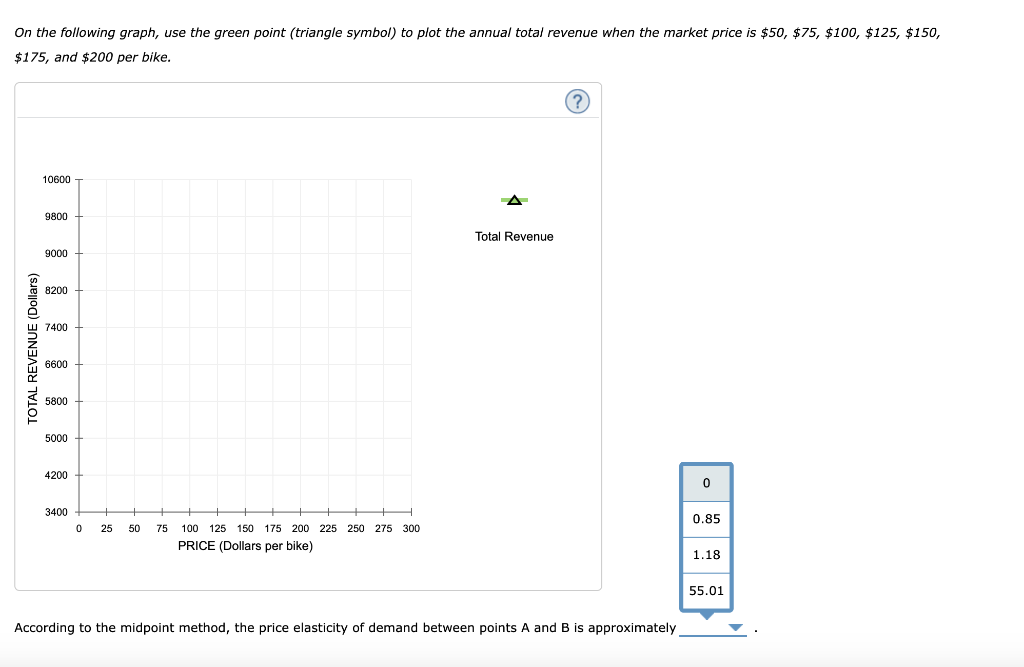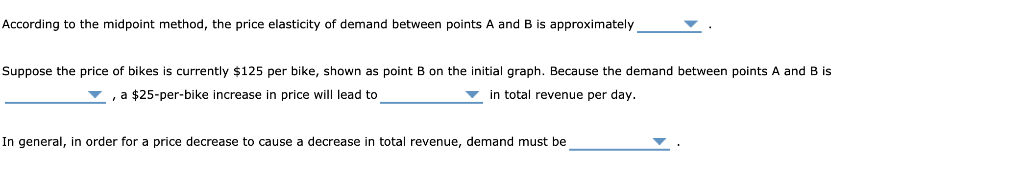### Create an Account

Home / Questions / Suppose the price of bikes is currently \$125 per bike, shown as point B on the initial gra...

# Suppose the price of bikes is currently \$125 per bike, shown as point B on the initial graph. BecausSuppose the price of bikes is currently \$125 per bike, shown as point B on the initial graph. Because the demand between points A and B is ELASTIC OR INELASTIC OR UNIT ELASTIC, a \$25-per-bike increase in price will lead to A DECREASE OR INCREASE OR NO CHANGE in total revenue per day.

n general, in order for a price decrease to cause a decrease in total revenue, demand must be  ELASTIC OR INELASTIC OR UNIT ELASTIC .

Use the green rectangle (triangle symbols) to compute total revenue at various prices along the demand curve. Note: You will not be graded on any changes made to this graph. 300 275 250 Total Revenue 225 200 175 A 150 125 100 75 50 25 Demand 0 C 10 20 30 40 50 60 70 80 90 100 110 120 QUANTITY (Bikes). PRICE (Dollars per bike) On the following graph, use the green point (triangle symbol) to plot the annual total revenue when the market price is \$50, \$75, \$100, \$125, \$150, \$175, and \$200 per bike. 10600 9800 Total Revenue 9000 8200 u 7400 6600 5800 5000 4200 0 3400 0.85 0 100 125 150 175 200 225 250 275 300 25 50 75 PRICE (Dollars per bike) 1.18 55.01 According to the midpoint method, the price elasticity of demand between points A and B is approximately 8(Dolars) According to the midpoint method, the price elasticity of demand between points A and B is approximately Suppose the price of bikes is currently \$125 per bike, shown as point B on the initial graph. Because the demand between points and B is in total revenue per day. \$25-per-bike increase in price will lead to In general, in order for a price decrease to cause decrease in total revenue, demand must be

Apr 03 2020 View more View LessSubscribe To Get Solution